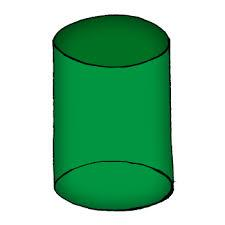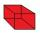# Surface of the cylinder

Calculate the surface area of the cylinder when its volume is 45 l and the perimeter of base is three times of the height.

S =  70.0527 dm2

### Step-by-step explanation:Did you find an error or inaccuracy? Feel free to write us. Thank you!Tips to related online calculators
Do you know the volume and unit volume, and want to convert volume units?

## Related math problems and questions:

• Cylinder - areaThe diameter of the cylinder is one-third the length of the height of the cylinder. Calculate the surface of cylinder if its volume is 2 m3.
• Perimeter of baseThe circumference of the base of the rotating cylinder is same as its height. What is the diameter and height of this cylinder with volume 1 liter?
• Equilateral cylinderEquilateral cylinder (height = base diameter; h = 2r) has a volume of V = 199 cm3 . Calculate the surface area of the cylinder.
• CylinderCalculate the dimensions of rotating cylindrical containers with volume 2 l if the container's height is equal to the base's diameter.
• Cube-shaped boxThe cube-shaped box is filled to the brim with 2 liters of milk. Calculate the edge and surface of the box.
• Cylinder surface, volumeThe area of the cylinder surface and the cylinder jacket are in the ratio 3: 5. The height of the cylinder is 5 cm shorter than the radius of the base. Calculate surface area and volume of the cylinder.
• CubeOne cube has an edge increased five times. How many times will larger it's surface area and volume?
• Volume and surfaceCalculate the volume and surface area of the cylinder when the cylinder height and base diameter is in a ratio of 3:4 and the area of the cylinder jacket is 24 dm2.
• Magnified cubeIf the lengths of the cube's edges are extended by 5 cm, its volume will increase by 485 cm3. Determine the surface of both the original and the magnified cube.
• Area of a cubeCalculate the surface area of a cube if its volume is equal to 729 cubic meters.
• Special cubeCalculate the edge of cube, if its surface and its volume is numerically equal number.
• Chemical parisonThe blown parison (with shape of a sphere) have a volume 1.5 liters. What is its surface?
• Cube surfce2volumeCalculate the volume of the cube if its surface is 150 cm2.
• Body diagonalThe cuboid has a volume of 32 cm3. Its side surface area is double as one of the square bases. What is the length of the body diagonal?
• Diameter = heightThe cylinder's surface, the height of which is equal to the diameter of the base, is 4239 cm square. Calculate the cylinder volume.
• The cubeThe cube has a surface area of 486 m ^ 2. Calculate its volume.
• Area to volumeIf the surface area of a cube is 486, find its volume.